# Kedlaya: Counting Points using p-adic Cohomology

We introduce a different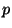-adic setup for counting points (or equivalently computing zeta functions). If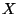is a variety over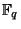, we want to count points using de Rham' cohomology. We will demonstrate Monsky-Washnitzer cohomology (a kind of rigid cohomology for smooth affine varieties). We restrict to the case whereis an affine curve, since in higher dimensions other methods will be faster. Then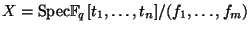.

Let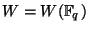be the Witt vectors, and let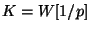be the fraction field of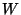. Define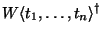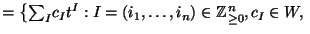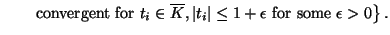In other words,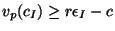for some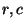with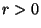. Modulo,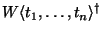reduces to the polynomial ring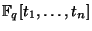, since all but finitely many coefficients are divisible by. We define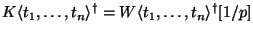. Let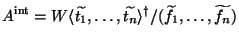whereis a lift of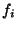. Then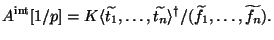There exists a lift so that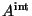is flat over.

Let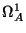be the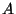-module generated by symbols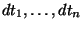modulo the submodule generated by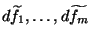. Then there is a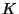-linear derivation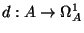. Letting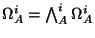; you get the de Rham complex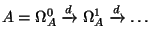and you define'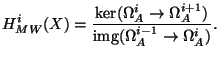It turns out that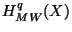is independent of choices (is unique up to noncanonical isomorphism, funny automorphisms are homotopic to the identity) and given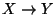, there is a map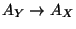and the induced maps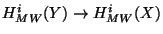also do not depend on choices.

The spaces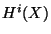are finite-dimensional, but it is not obvious; it relies upon relating this cohomology to rigid cohomology for proper varieties, namely, crystalline cohomology which we know is finite-dimensional for other reasons. Moreover, they satisfy the Lefschetz trace formula: if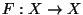is the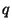-power Frobenius, then (Monsky)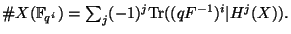The idea: try to computeand the map induced by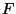(find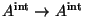lifting-power Frobenius).

Example. Look at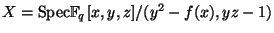with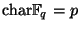odd. Let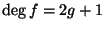,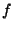monic. Lift it to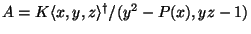, where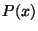is monic, degree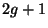over. It is easy to compute that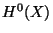is one-dimensional. Now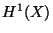is generated by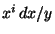for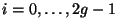, and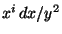,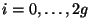. Notesplits under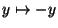into plus and minus eigenspaces.

You need to find relations inthat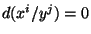. (This is a special situation: all relations are `algebraic'.) Lift the-power Frobenius by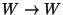by the Witt vector Frobenius,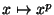, and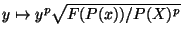. Compose this map with itself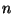times to get a-power Frobenius lift, and this allows us to compute the zeta function of a genus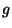hyperelliptic curve over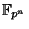in time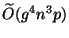.

Back to the main index for Future directions in algorithmic number theory.